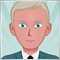Is it right that indicators can only work at ‘bar’ level, not at ‘tick’ level?686

Hi,

Is it right that indicators can only work at ‘bar’ level, not at ‘tick’ level?

thanksModerator
17041

Jose Luis Lominchar:

Hi,

Is it right that indicators can only work at ‘bar’ level, not at ‘tick’ level?

thanks

They can work at tick level in real time, but not historically.11565

Jose Luis Lominchar:

Hi,

Is it right that indicators can only work at ‘bar’ level, not at ‘tick’ level?

thanks

By default indicators' routine occurs on bars, but one can tweak an indicator to process each ticks.686

Thank you both.

Icham, can you provide some detail on how that tweak could be done?11565

Jose Luis Lominchar:

Thank you both.

Icham, can you provide some detail on how that tweak could be done?

Yeah. Very simply.

```int OnCalculate(const int rates_total,
const int prev_calculated,
const int begin,
const double &price[])
{ <------ even if this occurs each ticks
//--- check for data
if(rates_total<2*InpPeriodEMA-2)
return(0);
//---
int limit;
if(prev_calculated==0)
limit=0;
else limit=prev_calculated-1;
//--- calculate EMA
ExponentialMAOnBuffer(rates_total,prev_calculated,0,InpPeriodEMA,price,Ema);
//--- calculate EMA on EMA array
ExponentialMAOnBuffer(rates_total,prev_calculated,InpPeriodEMA-1,InpPeriodEMA,Ema,EmaOfEma);
//--- calculate DEMA
for(int i=limit;i<rates_total && !IsStopped();i++)
DemaBuffer[i]=2*Ema[i]-EmaOfEma[i]; <---- each element of this array is a bar
//--- OnCalculate done. Return new prev_calculated.
return(rates_total);
}```686

Icham Aidibe:

Yeah. Very simply.

Its looks great! thank you very much!11565

Jose Luis Lominchar:

Its looks great! thank you very much!

You welcome. Keep in mind that ...

Calculation based on data array

 int  OnCalculate(    const int        rates_total,       // price[] array size    const int        prev_calculated,   // number of handled bars at the previous call    const int        begin,             // index number in the price[] array meaningful data starts from    const double&    price[]            // array of values for calculation    );

Calculations based on the current timeframe timeseries

 int  OnCalculate(    const int        rates_total,       // size of input time series    const int        prev_calculated,   // number of handled bars at the previous call    const datetime&  time{},            // Time array    const double&    open[],            // Open array    const double&    high[],            // High array    const double&    low[],             // Low array    const double&    close[],           // Close array    const long&      tick_volume[],     // Tick Volume array    const long&      volume[],          // Real Volume array    const int&       spread[]           // Spread array    );

All these arrays are bars. So you have to gather ticks datas yourself.